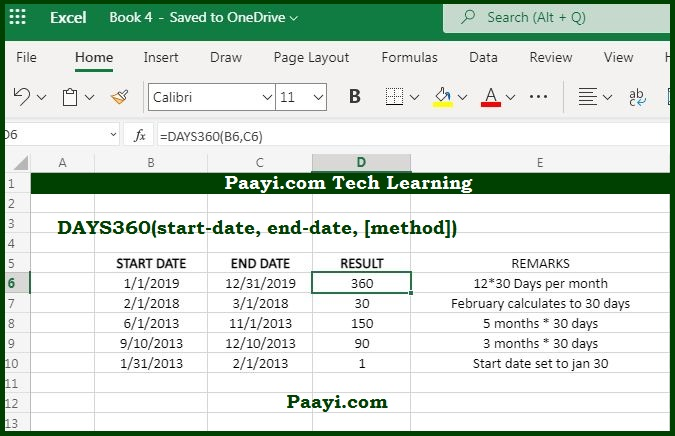# Learn How to Use Microsoft Excel DAYS360 Function

Written by | 0 Comments | 718 Views

In this article, you will learn how to use the Microsoft Excel DAYS360 function and its prime function in Microsoft Excel. You will also get to know the Microsoft Excel DAYS360 function return value and syntax with the help of some examples.

## Microsoft Excel DAYS360 Function

The main purpose of the DAYS360 function is to get the days between two dates in a 360-day year. That means the Microsoft Excel DAYS360 function returns the total number of days between the two specified dates based on a 360-day year. The calculation is made keeping 360-days in a year, keeping in mind specific accounting calculations where every month is considered to have 30 days.

### DAYS360 Function Return Value

It returns a number representing days.

### DAYS360 Function Syntax

= DAYS360(start-date, end-date, [method])

Where:

• start-date: It is the starting date.
• end-date: It is the end date.
• method: It is optional and used as per the day count basis. FALSE default (US meth0d), TRUE is the European method.

## How to Use Microsoft Excel DAYS360 Function?#### The DAYS360 function can be used in two ways:

• FALSE (US Method): When the start date is the last date of the month, it is set to the 30th day of a similar month. In case the end date is the last date of the month & the start date is less than 30, in this case, the end date is set as the 1st of the following month; if not, the end date is set to the 30th of the similar month.
• TRUE (European Method): Here, the start date and the end date is equal to the 31st of the month are set to the 30th of the same month.